Tag: #Target SBI PO 2017# MATHEMATICS FORMULA BOOK

### This book contains approx. 1300 formulas that covers all sections of Quantitative aptitude.It will help to increase you speed as you know SBI Pre is all about speed and accuracy.

BEST WISHES!!!# ENGLISH BOOK PDF

Objective General English is essential for those who aspire to clear examinations like the Banking,MBA, Hotel Management, BEd and UPSC. It is divided into four sections:
• Part A – foundation module
• Part B – verbal ability section,
• Part C & D – practical grammar section
It has a detailed account of everything a student requires to ace any competitive exam. It has chapters based on comprehensive topics like Tenses, Sentence Formation, Phrases, Basic Grammar, Synonyms/Antonyms, Punctuation and Transformation.
This edition also contains many revision test papers, which test the knowledge of the students on previously covered topics. There are also answer keys at the end of the test papers, which ensure that the student has got the right answer to every question. The book is structured in a highly effective manner and it contains various writing activities, improving the candidate’s writing ability. Objective General English, created by S.P. Bakshi, is 735 pages of concepts and subjects revolving around general English, covering words and meanings, foreign words expressions, homonyms and more.
The book has been updated to include the revised pattern of multiple competitive examinations. The new section on practical grammar has topics like Narration, Spelling and Contraction Rules. The workbook exercises at the end are helpful to the process of learning, giving the candidate ample practice.

# Syllogism capsule pdf# SBI PO 2017 ALL IN ONE LINK DIGEST

DOWNLOAD : all study material based on latest pattern including quant,reasoning,english,mock tests,previous year papers,exercises & much more# PERCENTAGE

The quant. section includes many important topics like- percentage, average, simple and compound interest, time and work, time and distance, algebra etc. We provide you with a guide on how to solve percentage and average related problems.

A percentage is a number or ratio expressed as a fraction of 100.Percentage is considered a very important chapter.

1. Firstly remember tables from 1 to 30.
2. Remember reciprocals of 1 to 15.
3. Remember squares of 1 to 30.
4. Learn cube root up to 20
5. Square root Up to 15

When we say 100 percent in mathematical notation we write 100% so 35 % means 35 per 100 and 65% means 65 per 100 it is a proportion per hundred and it is used to find marks, profit percent or loss percent of a particular product.

Here are some different percent related problems given with shortcut tricks below.

Percentage is a fraction whose denominator is always 100. x percentage is represented by x%.

### To express x% as a fraction :

We know   x% = x/100
Thus 10% = 10/100 (means 10 parts out of 100 parts)  = 1/10 (means 1 part out of 10 parts)

### To express x/y as a percentage :

We know that x/y  = (x/y× 100 )

Thus 1/4 = ( 1/4 ×100 )% = 25%
and 0.8 = ( 8/10 ×100 )% = 80%

### If the price of a commodity increases by R%, then reduction in consumption as not to increase the expenditure is-

[ R/(100+R)×100 ] %

### If the price of a commodity decreases by R%, then the increase in consumption as not to decrease the expenditure is –

[ R/(100-R)×100 ] %

### Result on Population :

Let the population of a town be P now and suppose increases the rate of R% per annum, then :

1. Population after n years = P ( 1+ R/100 )n
2. Population  n years ago = P /( 1+ R/100 )n

### Result on Depreciation :

Let the present value of a machine be P. Suppose depreciates at the rate of R% per annum Then :
1. Value of the machine after n Years

= P ( 1- R/100 )n
2. Value of the machine n years ago
P /( 1- R/100 )n
• If A is R% more than B, then B is less than A by

[ R/(100+R)×100 ]%

If A is R% less than B, then B is more than A by
[ R/(100-R)×100 ]%
• Net % change = x + y + xy/100

### Some Observation   #1

If 20% candidate failed in an exam then observations are

• 80% represent passed in exam
• 100% represent total appeared in exam
• (80%-20%) = 60% represent difference between passed and failed candidate in exam

### #2

If a number is increased by 25% then observations are
• 100% represent the old number
• 125% represent the new number.

### #3

Remember that Base in the given sentence (Question) is always 100%
Eg. Income of Ram is increased by 20%
In this sentence
100% – represent the income of Ram
20% – represent increment
120% – represent new income of Ram.

1 = 100%
1/2 = 50%
1/3 = 33 1/3%
1/4 = 25%
1/5 = 20%
1/6 = 162/3%
1/7 = 142/7%
1/8 = 121/2%
1/9 = 111/3%
1/10 = 10%
1/11 = 91/11%
1/10 = 81/3%
1/13% = 79/13%
25% = 1/4
6.25% = 1/16
125% = 5/4
150% = 3/2
200% = 2
350% = 7/2

### #1

Q. If the difference between 62% of a number and 3/5th of that number is 36. what is the number ?
Sol:
Let the number be x.
Then x × 62% – x × 3/5 = 36
x ×62% -x V 60% = 36 (60% = 3/5)
x ×2% = 36
x ×2/100 =36
x = 36 ×100/2 = 1800

### #2

Q. 40% of Ram’s income Rs. 1200 Then Find
1. 75% of Ram’s income ?
2. 1/4 part of Ram’s income ?
3. 1/3 part of Ram’s income ?

Sol :

(1)
40% = 1200 Rs.
75% = 1200/40 ×75 = 2250 Rs.

#### Trick : 1200 / 40  × 75 = Rs. 2250/-

(2)
40% of income = Rs. 1200
Then 1/4 part (i.e. 25% ) of Ram’s
income = 1200/40 ×25
= Rs. 750/- Ans
(3)
40% of Ram’s income
= Rs. 1200
i.e. 2/5 part of Ram’s income
= Rs. 1200
Then total income of Ram
= Rs. 1200 ×5/2
1/3 part of Ram’s income
= Rs. 1200  × 5/2  × 1/3
= Rs. 1000 Ans.

#### Trick :

1200/2/5  × 1/3
= 1200/2 × 5/3 = 1000

## EXERCISE-1

Example 1: The average mark of 70 students in a class is 80. Out of these 70 students, if the average mark of 40 students is 75, what is the average mark of the remaining 30 students?

Average of 70 students = 80.
Hence total marks of all the 70 students = 70×80 = 5600

Out of these 70, average of 40 students = 75
Hence total marks of these 40 students = 40×75 = 3000.

So the total marks of the remaining 30 students = 5600-3000 = 2600
Hence the average of the remaining 30 students = 2600/30 = 86.66

Now there will be a serious difference in the time taken if the numbers given here are not multiples of 10. Just look at example 2 below:

Example 2: “Average of 75 students is 82, out of which average of 42 students is 79. What is the average of the remaining 33 students?

Let’s go back to Example 1

Given that average of 70 students is 80. This class is split into two groups of 40 and 30. I am sure you will agree with me that if the average of each of these groups is 80, then the average of the total group i.e. 70 students will also be 80.

But the first group of 40 students, instead of 80 they had an average of only 75. So definitely the average of the remaining 40 students must be greater than 80.

The loss incurred because of the first group must be compensated by the second group.

Let us look at the loss incurred because of the first group

We want them to have an average of 80, but they managed only 75. So we lost an average of 5 upon 40 students. So the loss in the sum = 40×5.

Now this loss of 40×5 must be compensated by our second group i.e. 30 students.

So their average must be not only the initial 80, but also the average meant to compensate the loss incurred because of the first group.

Hence the average of the remaining 30 students = 80 + (40×5)/30 = 80 + 20/3 = 86.66

Now let us look at Example 2 where the numbers are not comfortable.

Example 3: Average of 75 students is 82, out of which average of 42 students is 79.
What is the average of the remaining 33 students?

Average of 75 members = 82.

Two groups of 42 and 33 and we want each group to have an average of 82.

But the first group i.e. 42 students they had an average of 79.
We fell short by 3 in the average.
So the loss in the sum= 3×42.

So the average of the second group i.e. 33 students must be 82 + (3×42)/33 = 82+ 42/11 = 85.82

So folks it’s time to get into some more different situations that arise when we calculate averages.

Problem 1:

Let’s look at one more sum similar to the one discussed above.

The average height of 74 students in a class is 168 cm out of which 42 students had an average height of 170 cm. Find the average height of the remaining 32 students.

Solution:

Average of 74 students = 168.
Here we can observe that, in the case of the first group we had a gain.
Since the average of 42 students is 170 cm, the gain is 2 cm in the average upon 42 students.
So the gain in the sum = 42×2

Hence average of the remaining 32 students = 168 – (42×2)/32= 168-(21/8) = 165.37

Problem 2:

The average height of 40 students in a class is 172 cm. 30 students whose average height is 172.5 cm left the class and 40 students whose average height is 170.5 cm joined the class. Find the average height of the present class.

Solution:

Going back to the ideal situation, we want the average of the students leaving the class as well as joining the class to be 172 so that the average remains the same.

But it is given that the average of the 40 students leaving the class is 172.5 (more than 172).
So we will incur a loss of 0.5 cm in the average upon 30 students.
Hence the loss in the sum = 0.5x 30 = 15 cm

Also, since the average of the 40 students joining the class is 170.5 (less than 172) we will incur a loss in this case as well. The loss in the average is 1.5 cm upon 40 students.
Hence the loss in the sum = 1.5×40 = 60 cm

Thus the total loss in the sum = 75 cm. This loss will be shared by 50 students which is the present strength of the class.

Hence the average of the present class = 172 – 75/50 = 170.5 cm.# PERMUTATIONS & COMBINATIONS

Important Concepts and Formulas – Permutations and Combinations 1. Multiplication Theorem (Fundamental Principles of Counting) If an operation can be performed in m different ways and following which a second operation can be performed in n different ways, then the two operations in succession can be performed in m×n different ways.

2. Addition Theorem (Fundamental Principles of Counting)

If an operation can be performed in m different ways and a second independent operation can be performed in n different ways, either of the two operations can be performed in (m+n) ways.

3. Factorial Let n

be a positive integer. Then n factorial can be defined as
n!=n(n1)(n2)1

Examples   5!=5×4×3×2×1=120 3!=3×2×1=6

Special Cases   0!=1 1!=1

4. Permutations: Permutations are the different arrangements of a given number of things by taking some or all at a time.Examples  All permutations (or arrangements) that can be formed with the letters a, b, c by taking three at a time are (abc, acb, bac, bca, cab, cba)  All permutations (or arrangements) that can be formed with the letters a, b, c by taking two at a time are (ab, ac, ba, bc, ca, cb)

5. Combinations : Each of the different groups or selections formed by taking some or all of a number of objects is called a combination.Examples Suppose we want to select two out of three girls P, Q, R. Then, possible combinations are PQ, QR and RP. (Note that PQ and QP represent the same selection.)

Suppose we want to select three out of three girls P, Q, R. Then, only possible combination is PQR

6. Difference between Permutations and Combinations and How to identify them

Sometimes, it will be clearly stated in the problem itself whether permutation or combination is to be used. However if it is not mentioned in the problem, we have to find out whether the question is related to permutation or combination.

Consider a situation where we need to find out the total number of possible samples of two objects which can be taken from three objects P, Q, R. To understand if the question is related to permutation or combination, we need to find out if the order is important or not.

If order is important, PQ will be different from QP, PR will be different from RP and QR will be different from RQ .If order is not important, PQ will be same as QP, PR will be same as RP and QR will be same as RQ. Hence,If the order is important, problem will be related to permutations.  If the order is not important, problem will be related to combinations.

For permutations, the problems can be like “What is the number of permutations the can be made”, “What is the number of arrangements that can be made”, “What are the different number of ways in which something can be arranged”, etc.

For combinations, the problems can be like “What is the number of combinations the can be made”, “What is the number of selections the can be made”, “What are the different number of ways in which something can be selected”, etc. pq and qp are two different permutations, but they represent the same combination.

Mostly problems related to word formation, number formation etc will be related to permutations. Similarly most problems related to selection of persons, formation of geometrical figures, distribution of items (there are exceptions for this) etc will be related to combinations.

7. Repetition

The term repetition is very important in permutations and combinations. Consider the same situation described above where we need to find out the total number of possible samples of two objects which can be taken from three objects P, Q, R.

If repetition is allowed, the same object can be taken more than once to make a sample. i.e., PP, QQ, RR can also be considered as possible samples.

If repetition is not allowed, then PP, QQ, RR cannot be considered as possible samples.

Normally repetition is not allowed unless mentioned specifically.

8. Number of permutations of n distinct things taking r at a time : Number of permutations of n distinct things taking r at a time can be given by

nPr = n!(nr)!

=n(n1)(n2)...(nr+1) where 0rn

Special Cases   nP0 = 1          nPr = 0 for r>n

nPr is also denoted by P(n,r). nPr has importance outside combinatorics as well where it is known as the falling factorial and denoted by (n)r or nr

Examples  8P2 = 8 × 7 = 56
5P4= 5 × 4 × 3 × 2 = 120

9. Number of permutations of n distinct things taking all at a time

Number of permutations of n distinct things taking them all at a time
= nPn = n!

10. Number of Combinations of n distinct things taking r at a time

Number of combinations of n distinct things taking r at a time ( nCr) can be given by
nCr = n!(r!)(nr)!

=n(n1)(n2)(nr+1)r! where 0rn

Special Cases   nC0 = 1,                  nCr = 0 for r>n

nCr is also denoted by C(n,r). nCr occurs in many other mathematical contexts as well where it is known as binomial coefficient and denoted by (nr)

Examples 8C2 = 8×7/2×1= 28,     5C4= 5×4×3×2/4×3×2×1= 5

Here’s an easy way to remember: permutation sounds complicated, doesn’t it? And it is. With permutations, every little detail matters. Alice, Bob and Charlie is different from Charlie, Bob and Alice (insert your friends’ names here).

Combinations, on the other hand, are pretty easy going. The details don’t matter. Alice, Bob and Charlie is the same as Charlie, Bob and Alice.

Permutations are for lists (order matters) and combinations are for groups (order doesn’t matter).

A joke: A “combination lock” should really be called a “permutation lock”. The order you put the numbers in matters. (A true “combination lock” would accept both 10-17-23 and 23-17-10 as correct.)

## Permutations: The hairy details

Let’s start with permutations, or all possible ways of doing something. We’re using the fancy-pants term “permutation”, so we’re going to care about every last detail, including the order of each item. Let’s say we have 8 people:

1: Alice
2: Bob
3: Charlie
4: David
5: Eve
6: Frank
7: George
8: Horatio


How many ways can we award a 1st, 2nd and 3rd place prize among eight contestants? (Gold / Silver / Bronze)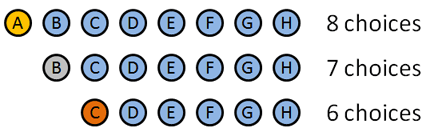We’re going to use permutations since the order we hand out these medals matters. Here’s how it breaks down:

• Gold medal: 8 choices: A B C D E F G H (Clever how I made the names match up with letters, eh?). Let’s say A wins the Gold.
• Silver medal: 7 choices: B C D E F G H. Let’s say B wins the silver.
• Bronze medal: 6 choices: C D E F G H. Let’s say… C wins the bronze.

We picked certain people to win, but the details don’t matter: we had 8 choices at first, then 7, then 6. The total number of options was 8 · 7 · 6 = 336.

Let’s look at the details. We had to order 3 people out of 8. To do this, we started with all options (8) then took them away one at a time (7, then 6) until we ran out of medals.

We know the factorial is: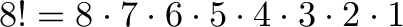$\displaystyle{ 8! = 8 \cdot 7 \cdot 6 \cdot 5 \cdot 4 \cdot 3 \cdot 2 \cdot 1 }$

Unfortunately, that does too much! We only want 8 · 7 · 6. How can we “stop” the factorial at 5?

This is where permutations get cool: notice how we want to get rid of 5 · 4 · 3 · 2 · 1. What’s another name for this? 5 factorial!

So, if we do 8!/5! we get: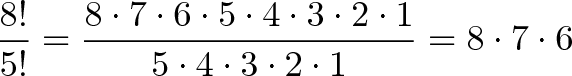$\displaystyle{\frac{8!}{5!} = \frac{8 \cdot 7 \cdot 6 \cdot 5 \cdot 4 \cdot 3 \cdot 2 \cdot 1}{5 \cdot 4 \cdot 3 \cdot 2 \cdot 1} = 8 \cdot 7 \cdot 6}$

And why did we use the number 5? Because it was left over after we picked 3 medals from 8. So, a better way to write this would be: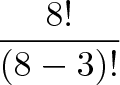$\displaystyle{\frac{8!}{(8-3)!}}$

where 8!/(8-3)! is just a fancy way of saying “Use the first 3 numbers of 8!”. If we have n items total and want to pick k in a certain order, we get: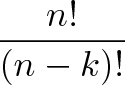$\displaystyle{\frac{n!}{(n-k)!}}$

And this is the fancy permutation formula: You have n items and want to find the number of ways k items can be ordered: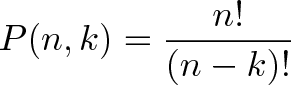$\displaystyle{P(n,k) = \frac{n!}{(n-k)!}}$

## Combinations, Ho!

Combinations are easy going. Order doesn’t matter. You can mix it up and it looks the same. Let’s say I’m a cheapskate and can’t afford separate Gold, Silver and Bronze medals. In fact, I can only afford empty tin cans.

How many ways can I give 3 tin cans to 8 people?

Well, in this case, the order we pick people doesn’t matter. If I give a can to Alice, Bob and then Charlie, it’s the same as giving to Charlie, Alice and then Bob. Either way, they’re equally disappointed.

This raises an interesting point — we’ve got some redundancies here. Alice Bob Charlie = Charlie Bob Alice. For a moment, let’s just figure out how many ways we can rearrange 3 people.

Well, we have 3 choices for the first person, 2 for the second, and only 1 for the last. So we have 3 · 2 · 1 ways to re-arrange 3 people.

Wait a minute… this is looking a bit like a permutation! You tricked me!

Indeed I did. If you have N people and you want to know how many arrangements there are for all of them, it’s just N factorial or N!

So, if we have 3 tin cans to give away, there are 3! or 6 variations for every choice we pick. If we want to figure out how many combinations we have, we just create all the permutations and divide by all the redundancies. In our case, we get 336 permutations (from above), and we divide by the 6 redundancies for each permutation and get 336/6 = 56.

The general formula is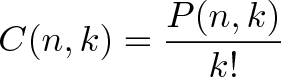$\displaystyle{C(n,k) = \frac{P(n,k)}{k!}}$

which means “Find all the ways to pick k people from n, and divide by the k! variants”. Writing this out, we get our combination formula, or the number of ways to combine k items from a set of n:$\displaystyle{C(n,k) = \frac{n!}{(n-k)!k!}}$

## A few examples

Here’s a few examples of combinations (order doesn’t matter) from permutations (order matters).

• Combination: Picking a team of 3 people from a group of 10. C(10,3) = 10!/(7! · 3!) = 10 · 9 · 8 / (3 · 2 · 1) = 120.

Permutation: Picking a President, VP and Waterboy from a group of 10. P(10,3) = 10!/7! = 10 · 9 · 8 = 720.

• Combination: Choosing 3 desserts from a menu of 10. C(10,3) = 120.

Permutation: Listing your 3 favorite desserts, in order, from a menu of 10. P(10,3) = 720.

Don’t memorize the formulas, understand why they work. Combinations sound simpler than permutations, and they are. You have fewer combinations than permutations.